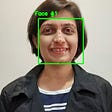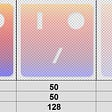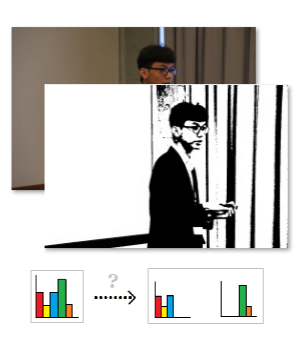Image by HBY coding academic | I'll be trying to reveal the secrets of Otsu thresholding in a mathematical way.

# Otsu Thresholding — Mathematical Secrets behind Image Binarization

Simple yet effective method – Otsu thresholding (envisioned in 1979) makes the conversion from grayscale to a binary image. It is based on the observation of the grayscale histogram and used only the zeroth- and first-order cumulative moment.

Even though this approach was proposed for over 40 years, it is still powerful to cover several practical applications. Interestingly, Otsu thresholding is everywhere, for example, widely used in those image binarization functions in Python or Matlab. In the article, I try to use easy-to-understand mathematical-supported examples to show you why Otsu thresholding makes sense.

## + How Otsu thresholding does?

Given an 8-bit grayscale image I, and the pixel value Pi of the i-th pixel, grayscale histogram H can be created by the number of occurrences of pixel value [0, 255]. Otsu thresholding directly adopts this statistical data to find the optimal cut t* that gives the best separation of the classes (for image binarization, foreground, and background).

Algorithmically, first establish the histogram H (see the above illustration, colored in blue). Second, for each threshold t in [0, 255], pixels can be separated into two classes, C1 and C2; those pixels whose Pi < t are put into C1, otherwise into C2. Then, we know the possibilities of C1 and C2 separated by t, denoted as W1 and W2, respectively. For example, W1 = (#pixels in C1) / (total pixels count). Third, given H, W1, and W2, for each t, compute the between-class variance Vb or within-class variance Vw (see the above illustration, it computes Vb and draws as a red curve). Finally, the optimal cut t* corresponds to t whose Vb is maximum or Vw is minimum.

## + Why do Otsu thresholding make sense

It can be explained in the following three different ways.

well-thresholded classes would be separated in gray levels, and conversely, a threshold giving the best separation of classes in gray levels would be the best threshold.

From this standpoint stated in the original paper , the author introduces “class separability” (CS, for short) to evaluate the goodness of the thresholding result.

(0) Higher CS
The higher the better. Why? Leave for you to think, and I will explain in the next section.

Either to minimize within-class variance Vw or to maximize between-class variance Vb can make foreground and background well-separated. Why?

(1) Maximum Vb
Given three classes C1, C2, and C3 contains black (grayscale value = 0), gray (grayscale value = 128), and white color (grayscale value = 255), respectively. Why does C1 look more different to C3 than to C2? Exactly due to the grayscale value difference between the two classes (at this point, you can think of it as Vb). Suppose one class represents the foreground and the other one is the background. Which two classes are the result that we expect? C1 and C3, since they are of the most visual difference between the two classes. That is why we prefer higher Vb for binarization.

(2) Minimum Vw
Given two separation cases, one is C1: pure black and C2:pure white, and the other is C1 and C2 with gray added. Which separation is the result we expect (can be visually distinguished one from the other without efforts)? The former, since each element is more identical to others in the same class than those in the latter one. In other words, the total variance of elements in C1 and C2 (denoted as V1 and V2) in the former case is smaller than those in the latter one (at this point, you can think of V1+V2 as Vw). Here comes the reason that the lower Vb is the better choice for binarization.

## + Let’s go deeper

In this section, we talk about the connection between “class separability” (CS, for short) and Vb (and Vw). Take a look at the following illustration.

Why do the classes on the left-hand side be in good separability? Two main reasons: (1) the classes (blue points, and yellow points) are farther from each other, and (2) every element in each class is most concentrated. Interestingly, as mentioned in the previous section, (1) implies maximum Vb, and (2) implies minimum Vw.

The term CS is from Fisher’s linear discriminant analysis. Otsu thresholding can be seen as a discrete version of CS and is equivalent to Vb/Vw. Recall that higher CS implies better thresholding results. Therefore, the larger Vb and smaller Vw, consistent with reasons (1) and (2), can make the value CS larger.

Since variance either lies between or within the class, the total variance Vt is held fixed (i.e., Vt = Vb + Vw). We can rewrite Vb/Vw as Vb/(Vt-Vb) or (Vt-Vw)/Vw. Observed that the larger Vb has the same contribution to the smaller Vw in that equation, and thus either one of the two reasons (1) and (2) can yield higher CS.

## + What are the mathematical expressions of Vb and Vw?

So far, we know why the best cut for image binarization in Otsu thresholding lies on the threshold t whose Vb is maximum (Vw is minimum). Do you curious about what Vw and Vb exactly are? To the best of my knowledge, little online material is talking about how to derive Vb and Vw. Now, let’s back to the image domain. You may have seen the equation, Vw = W1 ×V1 + W2 × V2.

Here, I expand each term by their definitions where N is the total pixel count in I, and W1 and W2 are the probabilities of the two classes C1 and C2 separated by t. C1 contains pixels with values less than t, while C2 contains those pixels which are not in C1. Since the size (pixel count) of C1 might be different from that of C2, we have to convert V1+V2 (as mentioned in (2) Minimum Vw) to a weighted variance sum for Vw.

As for Vb, you may have seen the following two equations.

In the second equation, Vb is proportional to the difference between class representatives (as mentioned in (1) Maximum Vb). How to derive it? Why does it have a quadratic term? Unlike Vw, it is not straightforward to define the Vb in a math way. However, we can use Vt and Vw to do back derivation.

First, expand squared total mean as colored in red where -2 is the clue to complete a square (a-b)² = - 2ab + . Second, substitute the weighted sum of μ1 and μ2 for μt. Third, use W1+W2=1 to merge and arrange the expansion terms. With these, we can derive the two equations.

There are two observations from the second derived equation:

1. given fixed W1×W2, a large mean difference encourages larger Vb.
2. given fixed mean difference, maximum Vb happens at W1 = W2.

For the observations above, Vb forms a push-pull factor during maximization where a push between μ1and μ2 and a pull between W1and W2. Also, during threshold finding in Otsu binarization, a pull exists within each class to make elements concentrated (i.e., similar pixel values); and a push exists between the two classes to let one be away from the other (i.e., larger mean difference).

## + The gap between ideal and real

Up to now, we know Otsu thresholding makes the decision is based on the observation of statistical data — histogram. In an ideal case, the histogram of one image has a sharp valley between two peaks (foreground and background) where the optimal cut lies. However, most real images do not have such distribution and make it hard to figure out the true valley precisely. For nearly 40 years, various extensions have been proposed to alleviate this problem for better performance.

## + Takeaway

1. Otsu thresholding is a binarization approach that utilizes the grayscale histogram to find the best separation result.
2. The optimal threshold lies on the separation result with maximum between-class variance (Vb) or minimum within-class variance (Vw).
3. The separation result is the production of push-pull optimization that makes each class more concentrated while pushing classes to be far away from each other.

## + Reference

 Otsu, Nobuyuki. “A threshold selection method from gray-level histograms.” IEEE transactions on systems, man, and cybernetics 9.1 (1979): 62–66.

--

--

--

## More from HBY coding academic

Image Processing/Game Designing/Informative and Interesting

Love podcasts or audiobooks? Learn on the go with our new app.

## Review of Deep Learning Algorithms for Image Classification## New Magical Abilities: Clone datasets## What Is the Sci-kit Learn Library?## Hyper-parameter Optimization with Manual Gradient — Part I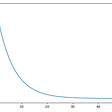## Intro to Support Vector Machines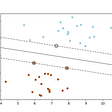## The Top ML/AI Python Packages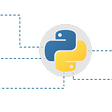## A Math-free Introduction to Machine LearningImage Processing/Game Designing/Informative and Interesting

## Understanding Calculus using NM Dev libraries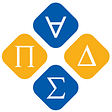## Deep learning text to speech (TTS) for noobs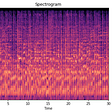## How to use facial landmarks obtained from dlib